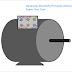There are different types of principles and methods available to generate electricity. Actually, electricity is a form of energy and we know that energy cannot be produced or destroyed we only can convert it. So, electricity also cannot be generated it is only converted from other energy sources. The generator is a device or machine that helps us to convert motive power(basically mechanical energy) into electrical energy. As casually, we can say we can generate electricity using a generator.

Basically, a generator uses electromagnetism, electromagnetic induction, Fleming's law, Faraday's law, etc to generate electricity.

A generator has main two parts - a Stator and a Rotor. Most of the generators are designed in such a way that when we rotate the rotor using an external prime mover the stator coil will generate electricity.

If we see the basic working principle of a generator is that when a changing magnetic field links with a conductor and magnetic flux cuts that conductor an EMF will be produced on that conductor. And this EMF will cause to flow the current through that conductor.

According to the type of energy source used to rotate the generator, electrical power generation plants are classified into different types.

For example, in a thermal power plant, thermal energy is used to produce steam from the water and this steam is used to rotate the generator with the help of a steam turbine. As the first input of this system is the thermal energy and the final output is electrical energy it is known as a thermal power plant.

The other types of power plants are - hydropower plants, nuclear power plants, and wind power plants. All of these power plants use electrical generators to generate electricity.

The electric motor is just opposite to an electric generator. An electric generator converts mechanical energy into electrical energy whereas an electric motor converts electrical energy into mechanical energy. Some electrical motors are there that can be used as a generator also. For example, a permanent magnet DC motor can be used as a generator also. If we rotate the rotor of that motor we can get electricity across its output terminals.

Now, coming to the nature of the electricity generated by a generator. It depends upon the type of generator. There are two types of generators. A DC generator can generate electricity in the form of direct current whereas an AC generator generates electricity in the form of alternating current.

Remember that a generator is basically a source of voltage not current. When they are connected to a closed circuit current starts flowing due to the voltage.

Basically, the DC generator and AC generator both work in the same principle. In the case of a DC generator, there is a commutator that converts the alternating current into the direct current so we can get the DC voltage at the output. However, synchronous AC generators and Induction generators work in a different principle than those DC generators.

In the case of a DC generator, there is no frequency concept. Here we just need to maintain the appropriate output voltage. But in the case of an AC generator, we have to maintain both the output frequency and voltage.

The output voltage of a generator depends upon so many constant and variable parameters. Some constant parameters are no.of turns in the stator winding, and magnetic field strength(for permanent magnet motor), and some variable parameters are Rotor speed, output load impedance, etc.

The output frequency of the generated power supply depends on two parameters - Motor RPM and the No. of Magnetic Poles. Magnetic poles we cannot change so we have to maintain the RPM of the motor to maintain the desired output frequency.

Here, the formula of generator frequency,

f=N*P/120

So, from this formula, we can say if the generator has two poles, it will provide a 60Hz output frequency at 3600RPM, or if the no. of poles is 4 then we need 1800 RPM.

Now, coming to the generator electricity cost. Actually, the cost of the generated electricity depends upon the types of sources of energy used as input energy, output load, and duration of use. For example, you can easily calculate the electricity cost of a diesel generator if know the diesel price. Just you have to measure how much diesel is consumed and then multiply it by its price.

Generator Electricity Principle, Nature, Types, Use, CostReviewed by Author on November 03, 2022 Rating: 5